So-Bogus A c++ sparse block matrix library aimed at Second Order cone problems
bogus::GaussSeidel< BlockMatrixType > Class Template Reference

Projected Gauss-Seidel iterative solver. More...

#include <GaussSeidel.hpp>

Inheritance diagram for bogus::GaussSeidel< BlockMatrixType >: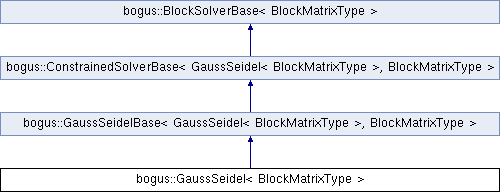## Public Types

typedef GaussSeidelBase
< GaussSeidel, BlockMatrixType >
Base

typedef Base::GlobalProblemTraits GlobalProblemTraits

typedef GlobalProblemTraits::Scalar Scalar

typedef LocalProblemTraits
< Base::BlockTraits::RowsPerBlock,
Scalar >
BlockProblemTraits

typedef BlockMatrixTraits
< BlockMatrixType >
BlockTraits

typedef Signal< unsigned, Scalar > CallBackType

## Public Member Functions

GaussSeidel ()
Default constructor – you will have to call setMatrix() before using the solve() function.

GaussSeidel (const BlockObjectBase< BlockMatrixType > &matrix)
Constructor with the system matrix.

GaussSeidelsetMatrix (const BlockObjectBase< BlockMatrixType > &matrix)
Sets the system matrix and initializes internal structures.

template<typename NSLaw , typename RhsT , typename ResT >
Scalar solve (const NSLaw &law, const RhsT &b, ResT &x, bool tryZeroAsWell=true) const
Finds an approximate solution for a constrained linear problem. More...

template<typename NSLaw , typename RhsT , typename ResT , typename LSDerived , typename HDerived >
Scalar solveWithLinearConstraints (const NSLaw &law, const BlockObjectBase< LSDerived > &Cinv, const BlockObjectBase< HDerived > &H, const Scalar alpha, const RhsT &b, const RhsT &c, ResT &x, bool tryZeroAsWell=true, unsigned solveEvery=1) const

template<typename NSLaw , typename RhsT , typename ResT , typename WDerived >
Scalar solveWithLinearConstraints (const NSLaw &law, const BlockObjectBase< WDerived > &W, const RhsT &b, ResT &x, bool tryZeroAsWell=true, unsigned solveEvery=1) const

Coloringcoloring ()
Access to the current Coloring. Will be reset whenever the matrix is changed. More...

void setMaxThreads (unsigned maxThreads=0)
Sets the maximum number of threads that the solver can use. More...

void setAutoRegularization (Scalar maxRegul)
Sets the auto-regularization (a.k.a. proximal point) coefficient. More...

void setEvalEvery (unsigned evalEvery)
Sets the number of iterations that should be performed between successive evaluations of the global error function. More...

void setSkipTol (Scalar skipTol)
Sets the minimum iteration step size under which local problems are temporarily frozen.

void setSkipIters (unsigned skipIters)
Sets the number of iterations for temporarily freezing local problems.

void useInfinityNorm (bool useInfNorm)
Sets whether the solver will use the infinity norm instead of the l1 one to compute the global residual from the local ones.

bool usesInfinityNorm () const

Scalar eval (const NSLaw &law, const ResT &y, const RhsT &x) const
Eval the current global residual as a function of the local ones. More...

void projectOnConstraints (const NSLaw &projector, VectorT &x) const
Projects the variable x on the constraints defined by projector.

void dualityCOV (const NSLaw &law, const RhsT &b, ResT &x) const
Compute associated change of variable (see NSLaw)

Scalar solve (const NSLaw &law, const RhsT &b, ResT &x) const

void setMaxIters (unsigned maxIters)
For iterative solvers: sets the maximum number of iterations.

unsigned maxIters () const

void setTol (Scalar tol)
For iterative solvers: sets the solver tolerance.

Scalar tol () const

CallBackTypecallback ()
Callback hook; will be triggered every N iterations, depending on the solver. More...

const CallBackTypecallback () const

const BlockObjectBase
< BlockMatrixType > &
matrix () const

## Protected Types

typedef Base::Index Index

typedef
Base::BlockProblemTraits::Matrix
DiagonalBlockType

## Protected Member Functions

void updateLocalMatrices ()

template<typename NSLaw , typename RhsT , typename ResT >
void innerLoop (bool parallelize, const NSLaw &law, const RhsT &b, std::vector< unsigned char > &skip, Scalar &ndxRef, ResT &x) const

const BlockMatrixBase
< BlockMatrixType > &
explicitMatrix () const

const IterableBlockObject
< BlockMatrixType > &
iterableMatrix () const

void processLocalMatrices ()

Scalar evalAndKeepBest (const NSLaw &law, const ResT &x, const typename GlobalProblemTraits::DynVector &y, typename GlobalProblemTraits::DynVector &x_best, Scalar &err_best) const

bool tryZero (const NSLaw &law, const RhsT &b, ResT &x, typename GlobalProblemTraits::DynVector &x_best, Scalar &err_best) const

void updateScalings ()

## Protected Attributes

Coloring m_coloring

ResizableSequenceContainer
< DiagonalBlockType >::Type
m_localMatrices

GlobalProblemTraits::DynVector m_regularization

unsigned m_maxThreads
See setMaxThreads(). Defaults to 0 .

unsigned m_evalEvery
See setEvalEvery(). Defaults to 25.

Scalar m_skipTol
See setSkipTol(). Defaults to 1.e-6.

unsigned m_skipIters
See setSkipIters() Defaults to 10.

Scalar m_autoRegularization

GlobalProblemTraits::DynVector m_scaling

bool m_useInfinityNorm
See useInfinityNorm(). Defaults to false.

const BlockObjectBase
< BlockMatrixType > *
m_matrix
Pointer to the matrix of the system.

unsigned m_maxIters
See setMaxIters()

Scalar m_tol
See setTol()

CallBackType m_callback

## Detailed Description

### template<typename BlockMatrixType> class bogus::GaussSeidel< BlockMatrixType >

Projected Gauss-Seidel iterative solver.

Works by taking into account only one block-row of the system at a time, and iterating several times over the whole set of rows several times until convergence has been achieved.

Each inner iteration of the algorithm will try to solve the local problemwhere k is the current global iteration, i the current row and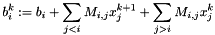See also solve() and .

## Member Function Documentation

template<typename BlockMatrixType >
 CallBackType& bogus::BlockSolverBase< BlockMatrixType >::callback ( )
inherited

Callback hook; will be triggered every N iterations, depending on the solver.

Useful to monitor the convergence of the solver. Can be connected to a function that takes an unsigned and a Scalar as parameters. The first argument will be the current iteration number, and the second the current residual.

See Also
Signal< unsigned, Scalar >
template<typename BlockMatrixType>
 Coloring& bogus::GaussSeidel< BlockMatrixType >::coloring ( )

Access to the current Coloring. Will be reset whenever the matrix is changed.

Determiniticy is achieved through the mean of contact coloring ; contacts that do not interact directly together can chare the same color, and all contacts within a given color can be solver in parallel

 Scalar bogus::ConstrainedSolverBase< GaussSeidel< BlockMatrixType > , BlockMatrixType >::eval ( const NSLaw & law, const ResT & y, const RhsT & x ) const
inherited

Eval the current global residual as a function of the local ones.

y should be such that y = m_matrix * x + rhs

Returns
the current residual err defined as follow :
• if m_useInfinityNorm is true, then err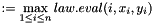• else err :=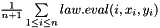void bogus::GaussSeidelBase< GaussSeidel< BlockMatrixType > , BlockMatrixType >::setAutoRegularization ( Scalar maxRegul )
inherited

Sets the auto-regularization (a.k.a. proximal point) coefficient.

The regularization works by slightly altering the local problems, so at each iteration we try to solvewhere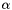is the regularization coefficient.

Note that as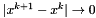when the algorithm converges, we are still trying to find a solution of the same global problem.

For under-determined problems, regularization might helps preventing x reaching problematically high values. Settingto a too big value will however degrade the convergence of the global algorithm.

Parameters
 maxRegul If greater than zero, then positive terms will be added to the diagonal of the local matrices so that their minimal eigenvalue become greater than maxRegul.
 void bogus::GaussSeidelBase< GaussSeidel< BlockMatrixType > , BlockMatrixType >::setEvalEvery ( unsigned evalEvery )
inherited

Sets the number of iterations that should be performed between successive evaluations of the global error function.

( Those evaluations require a full matrix/vector product, and are therfore quite costly )

 void bogus::GaussSeidelBase< GaussSeidel< BlockMatrixType > , BlockMatrixType >::setMaxThreads ( unsigned maxThreads = 0 )
inherited

Sets the maximum number of threads that the solver can use.

If \p maxThreads is zero, then it will use the current OpenMP setting.

Warning
If multi-threading is enabled without coloring, the result will not be deterministic, as it will depends on the order in which threads solve contacts.

On the other hand, the algorithm will run much faster.

template<typename BlockMatrixType>
template<typename NSLaw , typename RhsT , typename ResT >
 Scalar bogus::GaussSeidel< BlockMatrixType >::solve ( const NSLaw & law, const RhsT & b, ResT & x, bool tryZeroAsWell = true ) const

Finds an approximate solution for a constrained linear problem.

Stops when the residual computed in eval() is below m_tol, of the number of iterations exceed m_maxIters

Implements Algorithm 1. from  to solveParameters
 law The (non-smooth) law that should define: An error function for the local problem A local solver for each row of the system ( e.g. 1 contact solver )
See Also
SOCLaw
Parameters
 b the const part of the right hand side x the unknown. Can be warm-started tryZeroAsWell If true, the algorithm will reset r to zero if that would result in a lower residual
template<typename BlockMatrixType>
template<typename NSLaw , typename RhsT , typename ResT , typename LSDerived , typename HDerived >
 Scalar bogus::GaussSeidel< BlockMatrixType >::solveWithLinearConstraints ( const NSLaw & law, const BlockObjectBase< LSDerived > & Cinv, const BlockObjectBase< HDerived > & H, const Scalar alpha, const RhsT & b, const RhsT & c, ResT & x, bool tryZeroAsWell = true, unsigned solveEvery = 1 ) const

Solveswhere Cinv is such that Cinv * x =Warning
Requires: m_evalEvery multiple of solveEvery ;
template<typename BlockMatrixType>
template<typename NSLaw , typename RhsT , typename ResT , typename WDerived >
 Scalar bogus::GaussSeidel< BlockMatrixType >::solveWithLinearConstraints ( const NSLaw & law, const BlockObjectBase< WDerived > & W, const RhsT & b, ResT & x, bool tryZeroAsWell = true, unsigned solveEvery = 1 ) const

Solves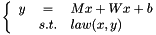with W arbitrary linear operator ( matrix or expression )

Warning
Requires: m_evalEvery multiple of solveEvery ;

## Member Data Documentation

 Scalar bogus::GaussSeidelBase< GaussSeidel< BlockMatrixType > , BlockMatrixType >::m_autoRegularization
protectedinherited
See Also
setAutoRegularization(). Defaults to 0.
template<typename BlockMatrixType>
 Coloring bogus::GaussSeidel< BlockMatrixType >::m_coloring
protected
See Also
coloring()

The documentation for this class was generated from the following file: